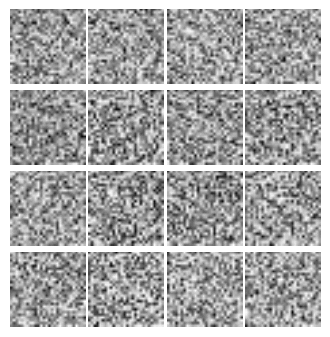## TensorFlow实现生成对抗网络GAN-翻译

2017年12月11日

### 生成对抗网络

◎ 一个成功的假币制造商会想尽方法骗过警察，使警察分不清假币与真币。

◎ 一个合格的警察会尽力分辨出假币和真币。

GAN是对抗过程的一个特例，它的组成（警察和假币制造商）是神经网络。第一个网络试图生成数据，第二个网络试图分辨出真实数据和第一个网络生成的伪造数据。第二个网络会输出表示真实数据概率的[0,1]之间的量。

$$\min_G \max_D {\mathbb E}_{x\sim p_{\rm data}} \log D(x) + {\mathbb E}_{z\sim p_z}[\log (1-D(G(z)))]$$

### GAN的实现

# Discriminator Net
X = tf.placeholder(tf.float32, shape=[None, 784], name='X')

D_W1 = tf.Variable(xavier_init([784, 128]), name='D_W1')
D_b1 = tf.Variable(tf.zeros(shape=), name='D_b1')

D_W2 = tf.Variable(xavier_init([128, 1]), name='D_W2')
D_b2 = tf.Variable(tf.zeros(shape=), name='D_b2')

theta_D = [D_W1, D_W2, D_b1, D_b2]

# Generator Net
Z = tf.placeholder(tf.float32, shape=[None, 100], name='Z')

G_W1 = tf.Variable(xavier_init([100, 128]), name='G_W1')
G_b1 = tf.Variable(tf.zeros(shape=), name='G_b1')

G_W2 = tf.Variable(xavier_init([128, 784]), name='G_W2')
G_b2 = tf.Variable(tf.zeros(shape=), name='G_b2')

theta_G = [G_W1, G_W2, G_b1, G_b2]

def generator(z):
G_h1 = tf.nn.relu(tf.matmul(z, G_W1) + G_b1)
G_log_prob = tf.matmul(G_h1, G_W2) + G_b2
G_prob = tf.nn.sigmoid(G_log_prob)

return G_prob

def discriminator(x):
D_h1 = tf.nn.relu(tf.matmul(x, D_W1) + D_b1)
D_logit = tf.matmul(D_h1, D_W2) + D_b2
D_prob = tf.nn.sigmoid(D_logit)

return D_prob, D_logit


discriminator(x)接受MNIST图片作为输入，返回一个代表真实图片概率的张量。G_sample = generator(Z)
D_real, D_logit_real = discriminator(X)
D_fake, D_logit_fake = discriminator(G_sample)

D_loss = -tf.reduce_mean(tf.log(D_real) + tf.log(1. - D_fake))
G_loss = -tf.reduce_mean(tf.log(D_fake))


# Only update D(X)'s parameters, so var_list = theta_D
D_solver = tf.train.AdamOptimizer().minimize(D_loss, var_list=theta_D)
# Only update G(X)'s parameters, so var_list = theta_G
G_solver = tf.train.AdamOptimizer().minimize(G_loss, var_list=theta_G)

def sample_Z(m, n):
'''Uniform prior for G(Z)'''
return np.random.uniform(-1., 1., size=[m, n])

for it in range(1000000):
X_mb, _ = mnist.train.next_batch(mb_size)

_, D_loss_curr = sess.run([D_solver, D_loss], feed_dict={X: X_mb, Z: sample_Z(mb_size, Z_dim)})
_, G_loss_curr = sess.run([G_solver, G_loss], feed_dict={Z: sample_Z(mb_size, Z_dim)})### 其他可选择的损失函数

# Alternative losses:
D_loss_real = tf.reduce_mean(tf.nn.sigmoid_cross_entropy_with_logits(D_logit_real, tf.ones_like(D_logit_real)))
D_loss_fake = tf.reduce_mean(tf.nn.sigmoid_cross_entropy_with_logits(D_logit_fake, tf.zeros_like(D_logit_fake)))
D_loss = D_loss_real + D_loss_fake
G_loss = tf.reduce_mean(tf.nn.sigmoid_cross_entropy_with_logits(D_logit_fake, tf.ones_like(D_logit_fake)))


### 参考文献